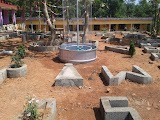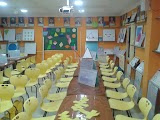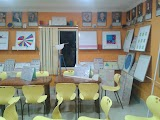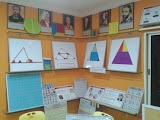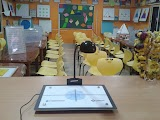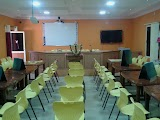# Mathematics Laboratory

Sometimes students may view mathematics as dull, boring or difficult. Students may think that mathematics means to ‘get something right’ or to ‘get something wrong’. When they get it wrong they think that they are not good enough for mathematics and lose interest in learning. On the other hand a few students learn enough tricks to ‘get something right’ to pass the exam. However when a teacher has tried to teach maths in a different way, say through activities and games, it can make the process of learning more interesting and effective. The setting up of a mathematics laboratory aids this effort.

A mathematics laboratory is a place where we find a collection of games, puzzles, teaching aids and other materials for carrying out activities. These are meant to be used both by the student by their own and together with their teacher to explore the world of mathematics, to discover, to learn and to develop an interest in mathematics. Although mathematics is not an experimental science in the way in which physics, chemistry and biology are, a mathematics laboratory can contribute greatly to the learning of mathematical concepts and skills.

# Activities in Mathematics Laboratory

## Project Ideas by Chapter

1. Measurement in Solid Figures
2. Algebraic Identities

# Need for a Mathematics Laboratory

1. Mathematics laboratory is activity centered and a child is placed in problem solving situation through self exploration and discovery. He provides a solution based on his experience, needs and interest. Some of the ways in which a mathematics laboratory can contribute to the learning of the subject are.
2. It provides an opportunity to understand and internalize the basic mathematical concepts through concrete objects and situations.
3. It enables the students to verify or discover several geometrical properties and facts using models or by paper cutting and folding technique.
4. It helps the students to build interest and confidence in learning the subject.
5. The laboratory provides opportunity to exhibit the relatedness of mathematical concepts with every day life.
6. It provides greater scope for individual participation in the process of learning and becoming autonomous learners.
7. It provides scope for greater involvement of both the mind and the hand which facilitate cognition.
8. The laboratory allows and encourages students to think, discuss with each other and the teacher and assimilates the concepts in a more effective manner.
9. It enables the teacher to demonstrate, explain and reinforce abstract mathematical ideas by using concrete objects, models, charts, graphs, pictures, posters etc.

# Objectives of Mathematics Laboratory

1. To provide readily accessible rich manipulative materials to emphasis on “learning by doing”.
2. To develop an attitude of enquiry.
3. Remove the weakness of present day mathematics education.
4. To develop much needed confidence in students.
5. To generate interest in the subject.
6. To make the students divergent thinkers.
7. To make the children to look for pattern and ask questions.

# Pictures of a Mathematics Laboratory